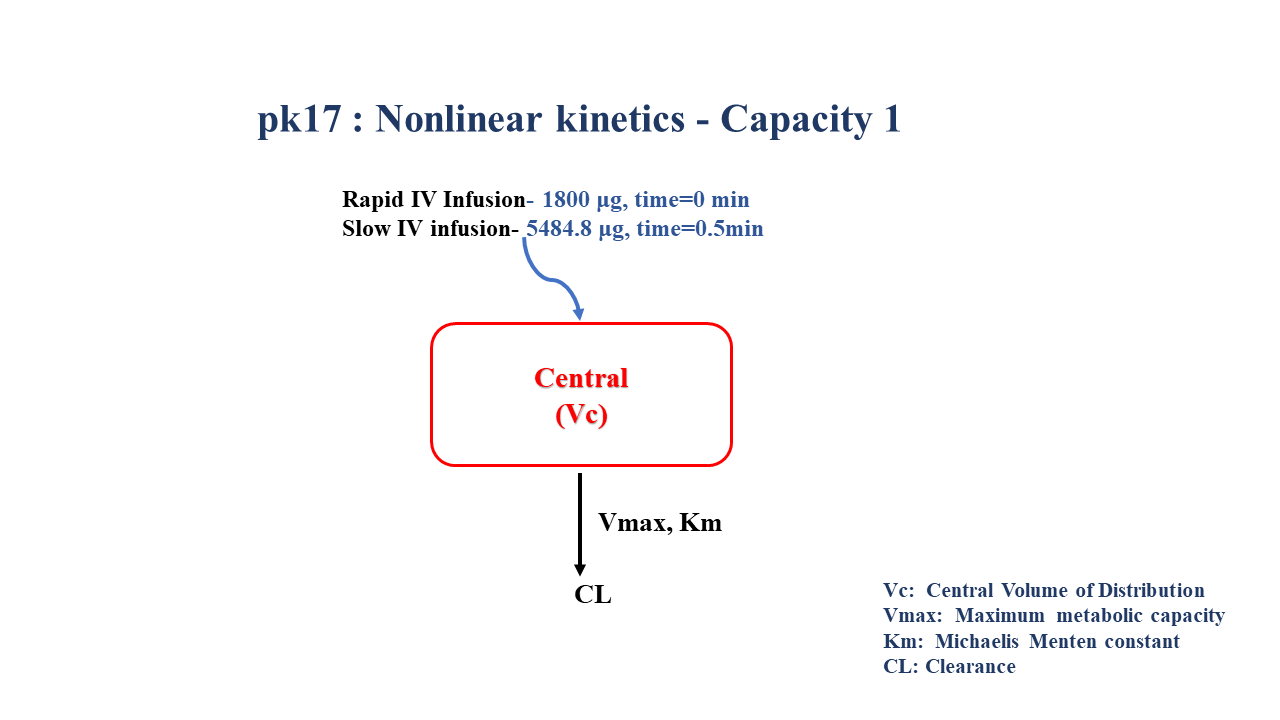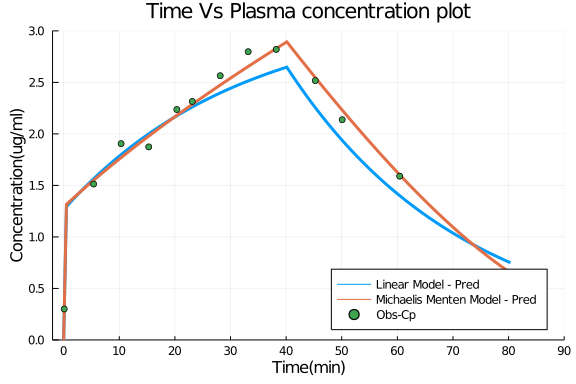# Exercise PK17 - Nonlinear kinetics - Capacity 1

### Background

Following info

• Structural model - One compartment non linear elimination

• Route of administration - IV infusion

• Dosage Regimen - 1800 μg Rapid IV, 5484.8 μg Slow IV

• Number of Subjects - 1### Learning Outcomes

In this model, you will learn -

• To build plasma concentration data following multiple intravenous infusions.

• To apply differential equation for one compartment model taking into consideration non linear elimination parameters.

• To simulate the dataset using final parameter estimates.

### Objectives

In this tutorial, you will learn how to build one compartment model for capacity limited kinetics and to simulate the model for single subject following multiple IV infusions.

### Libraries

Call the "necessary" libraries to get started.

using Pumas
using Plots
using CSV
using StatsPlots
using Random


### Model

In this One compartment model following linear elimination,multiple IV infusions are administered into the central compartment.

##### Linear Model
pk_17_lm     = @model begin
@param begin
tvcl     ∈ RealDomain(lower=0)
tvvc     ∈ RealDomain(lower=0)
Ω        ∈ PDiagDomain(2)
σ        ∈ RealDomain(lower=0)
end

@random begin
η        ~ MvNormal(Ω)
end

@pre begin
Cl       = tvcl   * exp(η)
Vc       = tvvc   * exp(η)
end

@dynamics begin
Central' = - (Cl/Vc)*Central
end

@derived begin
cp       = @. Central/Vc
dv       ~ @. Normal(cp,σ)
end
end

PumasModel
Parameters: tvcl, tvvc, Ω, σ
Random effects: η
Covariates:
Dynamical variables: Central
Derived: cp, dv
Observed: cp, dv

##### Parameters - Linear Model

The Parameters are as given below. tvrepresents the typical value for parameters.

• Cl - Clearance (ml/min)

• Vc - Volume of Distribution of Central Compartment (ml)

• Ω - Between Subject Variability

• σ - Residual Error

param_lm = (tvcl   = 43.3,
tvvc   = 1380 ,
Ω      = Diagonal([0.0, 0.0, 0.0]),
σ      =  0.00)

(tvcl = 43.3, tvvc = 1380, Ω = [0.0 0.0 0.0; 0.0 0.0 0.0; 0.0 0.0 0.0], σ =
0.0)

##### Michaelis Menten Model

In this One compartment model following nonlinear elimination,multiple IV infusions are administered into the central compartment.

pk_17_mm     = @model begin
@param begin
tvvmax   ∈ RealDomain(lower=0)
tvkm     ∈ RealDomain(lower=0)
tvvc     ∈ RealDomain(lower=0)
Ω        ∈ PDiagDomain(3)
σ        ∈ RealDomain(lower=0)
end

@random begin
η        ~ MvNormal(Ω)
end

@pre begin
Vmax     = tvvmax * exp(η)
Km       = tvkm   * exp(η)
Vc       = tvvc   * exp(η)
end

@dynamics begin
Central' = - (Vmax/(Km+(Central/Vc))) * (Central/Vc)
end

@derived begin
cp       = @. Central/Vc
dv       ~ @. Normal(cp,sqrt(cp^2*σ))
end
end

PumasModel
Parameters: tvvmax, tvkm, tvvc, Ω, σ
Random effects: η
Covariates:
Dynamical variables: Central
Derived: cp, dv
Observed: cp, dv

##### Parameters - Michaelis Menten Model

The Parameters are as given below. tvrepresents the typical value for parameters.

• Vmax - Maximum Metabolic Capacity (ug/min)

• Km - Michaelis-Menten Constant (ug/ml)

• Vc - Volume of Distribution of Central Compartment (ml)

• Ω - Between Subject Variability

• σ - Residual Error

param_mm = (tvvmax = 124.451,
tvkm   = 0.981806,
tvvc   = 1350.61 ,
Ω      = Diagonal([0.0, 0.0, 0.0]),
σ      =  0.005)

(tvvmax = 124.451, tvkm = 0.981806, tvvc = 1350.61, Ω = [0.0 0.0 0.0; 0.0 0
.0 0.0; 0.0 0.0 0.0], σ = 0.005)


### Dosage Regimen

A single subject received Rapid infusion of 1800 μg over 0.5 min followed by 5484.8 μg over 39.63 min.

DR= DosageRegimen([1800,5484.8], time=[0,0.5], cmt=[1,1], duration=[0.5,39.63])
s1= Subject(id=1, events=DR)

Subject
ID: 1
Events: 4


### Simulation

Lets simulate for plasma concentration for single subject for specific observation time points after mutiple IV infusion dosage.

Random.seed!(1234)
sim_lm = simobs(pk_17_lm, s1, param_lm, obstimes = 0.0:0.01:80.35)
Random.seed!(1234)
sim_mm = simobs(pk_17_mm, s1, param_mm, obstimes = 0.0:0.01:80.35)


### DataFrame & Plot

df_lm    = DataFrame(sim_lm)
dropmissing!(df_lm, :cp)
df_mm    = DataFrame(sim_mm)
dropmissing!(df_mm, :cp)
df_dv_mm = filter(x -> x.time in [0.1, 5.38, 10.33, 15.3, 20.35, 23.13, 28.15, 33.18, 38.23, 40.62, 45.25, 50.08, 60.42, 80.35],df_mm)

@df df_lm plot(:time, :cp, linewidth=3, legend=:bottomright,
label= "Linear Model - Pred", ylabel="Concentration(ug/ml)",
xlabel="Time(min)", title= ("Time Vs Plasma concentration plot"),
xticks=[0,10,20,30,40,50,60,70,80,90], xlims=(-2,90),
yticks=[0,0.5,1.0,1.5,2.0,2.5,3.0], ylims=(0,3.0))
@df df_mm plot!(:time, :cp, label="Michaelis Menten Model - Pred", linewidth=3)
@df df_dv_mm scatter!(:time, :dv, label= "Obs-Cp")